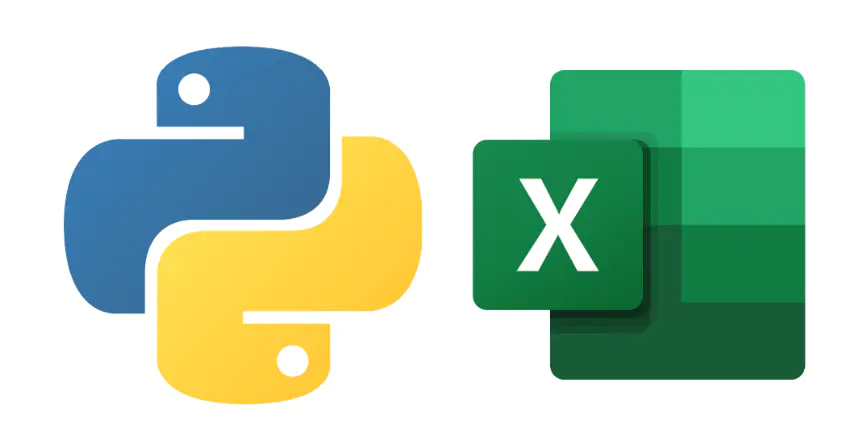# 一句代码合并Excel表### 1 读取表格

``````import pandas as pd
``````

``````import pandas as pd
``````

### 2 合并表格

`dataMerge=pd.merge(data1,data2,left_on='name',right_on='学员姓名',how='left')`

`dataMerge=pd.merge(data1,data2,on='name')`

### 3 保存表格

`dataMerge.to_excel('合并表格.xlsx')`

（版权归原作者所有，侵删）